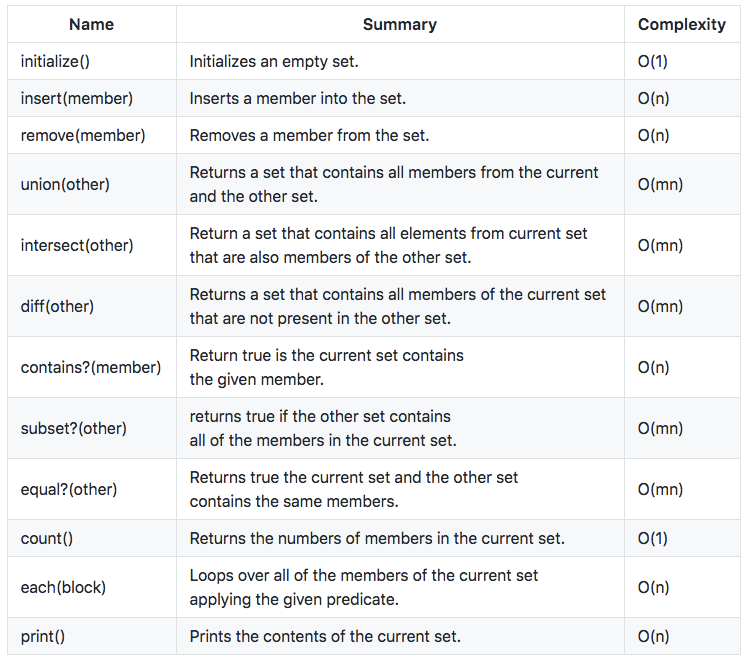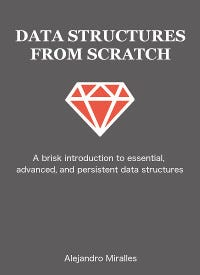# Mastering data structures in Ruby — Sets

A set is an unordered sequence of unique elements (called members) grouped because they related to each other in some way. Sets can contain other sets, can be empty, but they can’t contain duplicate members.

We can define sets by using sets notation:

``S = { 1, 2, 3 }``

A common way to describe sets is by their operations and properties:

#### Empty

A set with no elements is the empty set.

``{} => true``

#### Equal

Two sets are equal if they contain the same members. (Remeber that in the case of sets the order doesn’t matter.)

``{1, 2, 3} = {3, 2, 1} => true``

#### Subset

Set “a” is a subset of “b” if “b” contains all of the elements that are present in “a”.

``{3, 4} C {2, 3, 4} => true``

#### Union

A union of sets “a” and “b” produces a set “c” that contains all the elements from “a” and “b”.

``{1, 2, 3} U {4, 5, 6} => { 1, 2, 3, 4, 5, 6 }``

#### Intersect

An intersection of two sets produces a new set that contains only the elements that are present in both sets.

``{1, 2, 3} N {2, 3, 4} => { 2, 3 }``

#### Difference

A difference between two sets is a set that contains all the elements for the first set except the ones that are present in the second.

``{1, 2, 3} - {2, 3, 4} => { 1 }``

### Properties

• The intersection of a set with the empty set is the empty set.
• The union of set the “a” and the empty set is the set “a”.
• The intersection of a set with itself is the original set.
• The union of a set with itself is the original set.

There are a few more properties to sets but they are outside the scope I’m going to cover in this post.

Sets are widely used in math, probability, and combinatorics. All subjects equally interesting and way out of my league, so, I’ll focus on the set data structure instead.

The complexity of operations on sets is significantly different to what ‘ve seen on previous posts, mainly because:

• Most operations require to (potentially) traverse two sets O(mn).
• We have to guarantee no dupes which convert traditional O(1) operations, like inserts, into O(n) operations.

### Sets interface

What follows is a brief description of the set’s method and their complexity.Method names, summaries, and complexity.

### Implementation Details

To store set members, we are going to use the singly linked list we built in the first post of this series, which is nice because we get to reuse a lot working code that helps us to save a bunch of work.

We could also have use hash tables which would give us better insert/remove performance at a cost a bit slower full scans. As with most things in CS, it’s all about tradeoffs. In general, singly linked lists work better for small sets, though.

### Initialize

The only thing this method does is to initialize the set’s internal storage. The complexity of this method is O(1).

``def initialize    @list = LinkedList.newend``

### Insert

This method inserts a new member into the set. Since sets can’t contain duplicates, the first this method does is to check if the member is not already there. If it’s not, it inserts the new member otherwise it does nothing.

Since we have to check if the member is already there, the complexity of this method is O(n)

``def insert member    return if contains? member    @list.insert memberend``

### Remove

This method removes a member from the current set, or it does nothing if the element doesn’t exist.

Since we may have to walk the whole list the complexity of this method is O(n).

``def remove member    node = @list.find { |nd| nd.value == member }    @list.remove node if nodeend``

### Union

This method returns a set that contains all members of the current and the other set.

This operation involves walking two sets hence its complexity is O(mn).

``def union other    res = Set.new    @list.each { |nd| res.insert(nd.value) }    other.each { |nd| res.insert(nd.value) }    resend``

### Intersect

This method returns the intersection of the current set with the other set.

Since this operation involves walking two sets, its complexity is O(mn).

``def intersect other    res = Set.new    @list.each do |nd|         res.insert(nd.value) if other.contains?(nd.value)    end    resend``

### Diff

This method returns a set that contains all of the elements that are present in the current set but not in the other.

As well as it happens with union an intersect, this operation requires walking the two sets which make it O(mn).

``def diff other    res = Set.new    @list.each do |nd|         res.insert(nd.value) unless other.contains?(nd.value)    end    resend``

### Contains

This method returns true if the set includes the given member. To find a member this method walks the internal list and check each node data until it finds the member or the list runs out of nodes.

To complete this operation we might have to walk the whole list. Therefore its complexity is O(n).

``def contains? member    @list.find_first { |nd| nd.data == member }end``

### Subset

This method returns true if the current set is a subset of the other set and false otherwise.

In this case, we use two strategies: If the current set is larger than the other set, there is no way that if a subset of it. So we can take the expressway an exit earlier. The result is false.

If the previous condition is not true, we have to go the slow path instead and walk over all members in the current set.

Potentially, we may have to walk two sets; therefore the complexity of this method is O(mn).

``def subset? other    return false if self.count > other.count``
``@list.each do |nd|        return false unless other.contains(nd.value)    end    trueend``

### Equal

This method returns true if the current set is equal to the other set and false otherwise. As well as we did with the subset method, we do a quick check on set’s length first to see if we can exit fast if that check fails, we have to go the slow path instead.

As it happens with the subset method, we might have to walk two sets to complete this operation which makes its complexity O(mn).

``def equal? other    return false if self.count != other.count    subset? otherend``

### Count

This method returns the number of elements in the current set. The complexity of this method is O(1).

``def count    @list.lengthend``

### Each

This method walks the members in the current set yielding them one a time. The complexity of this method is O(n).

``def each    return nil unless block_given?``
``current = @list.head    while current        yield current&.data        current = current.next    endend``

### Print

This method prints the contents of the current set. The complexity of this method is O(n).

``def print    @list.printend``

### When to use sets

Sets are commonly used to data correlation. Or, in other words, to find relationships between different groups of data.

Also, as we will see in future posts, sets are used as a support data structure for graphs and graphs algorithms.

Of course, there is a whole lot more about sets, but that’s it for this brief introduction. I hope you enjoyed!

Next time, Binary Trees. Stay tuned.

As usual, you can get the source code from here.

Thanks for reading! And see you next time.

PS: Don’t forget to clap if you like list post :)

PS2: If you are a member of the Kindle Unlimited program, you can read a bit polished version of this series for free on your Kindle device.Data Structures From Scratch — Ruby Edition.